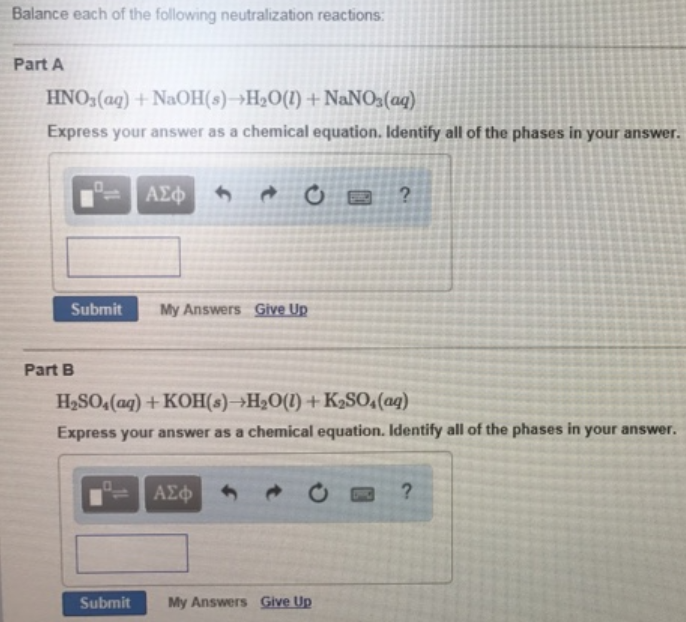# Problem: Balance each of the following neutralization reactions: Part A HNO3 (aq) + NaOH(s) → H2O(l) + NaNO3 (aq) Express your answer as a chemical equation. Identify all of the phases in your answer. Part B H2SO4 (aq) + KOH(s) → H2O(l) + K2SO4 (aq) Express your answer as a chemical equation. Identify all of the phases In your answer.

###### FREE Expert Solution
92% (378 ratings)###### Problem Details

Balance each of the following neutralization reactions:

Part A

HNO3 (aq) + NaOH(s) → H2O(l) + NaNO3 (aq)

Part B

H2SO4 (aq) + KOH(s) → H2O(l) + K2SO4 (aq)